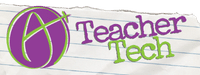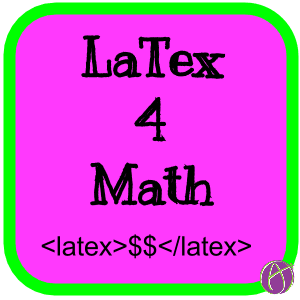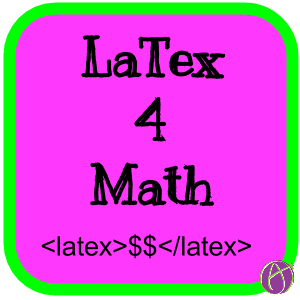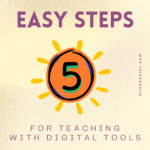# Math Teachers: Alice’s Guide to LaTex## Math Teachers: Alice’s Guide to LaTexDo not ask me how to pronounce LaTex, but as a math teacher it is a useful thing to know! In a nutshell LaTex let’s you put pretty print math symbols on the web. If you need fractions and square roots it can be very helpful to know just a little LaTex. Most websites will not recognize LaTex so try it out and see where you can use it. One reason I use www.quia.com is that I can use LaTex and HTML when I write questions. You can also use LaTex in Google Docs equation editor, but I find it easier to just use the equation editor templates.

This is just a quick intro to LaTex. You can find more expressions and tutorials on the web once you get these down. Check out http://mathx.co/ to type pretty print math expressions, but to also copy the LaTex code.

# <latex>$$</latex> The first thing I do is type <latex>$$</latex> when I am entering latex into Quia. Some Web 2.0 tools that accept LaTex do not need the <latex> </latex> and only require that you flank the equations with dollar signs. Other tools, like Google Docs equation editor, only need the LaTex expressions without the dollar signs. I find it helpful to type the end tag of </latex> (when it is required) before I type the LaTex expressions so that I do not accidentally forget it.

I also always type the beginning and ending curly brace before I type the values within the curly brace. This is especially helpful when you are nesting expressions. So I will type \sqrt{} and then fill in the radicand \sqrt{7} after I typed the generic expression.

# Backslash Forward Slash

In HTML the end tags have a forward slash </latex>. In LaTex the expressions use the backslash \frac{}. When your LaTex is not rendering properly, double check that your slashes are going the right way.

# Fraction

## \frac{}{}

Relatively intuitive is that the LaTex expression for creating a fraction is \frac{}{}. The curly braces contain the values for the numerator and the denominator. So if I want to type a fraction of two-thirds I would type:

<latex>$\frac{2}{3}$</latex>

# Square Root

## \sqrt{} or \sqrt[n]{}

Equally intuitive is the LaTex expression for creating a square root. Type \sqrt{} with the radicand in the curly braces. If you want the nth root you can place that value in square brackets before the curly braces. So the cube root of 7 would look like:

<latex>$\sqrt{7}$</latex>

I find I demonstrate the quadratic formula a lot for how to use LaTex so here is how you would type that:

<latex>$x=\frac{-b \pm \sqrt{b^2-4ac}}{2a}$</latex>

You can find the math symbols for things like the plus or minus on several websites. I usually do a search for LaTex math symbols and leave the tab open while I am working with LaTex.

# Try It Out

Hopefully this is enough to get you started. I find that most of the time I need fractions and square roots in my math problems. The other expressions and symbols I just look them up as I need them. So memorize those two and you’re pretty much good to go!

### Sample Quia Activities

I created these activities a long time ago. Notice that some of the questions contain fractions, square roots and other math symbols which required LaTex.

Complete the Square Millionaire

Fractions Jeopardy

Sets, Intersections and Unions Cloze Activity

Football Scrimmage### 5 Easy Steps for Teaching with Digital Tools

Introducing digital tools into your teaching can seem challenging at first. There are many tools out there, and it might feel like there’s a lot to learn. But with easy steps for teaching with digital tools, you can start simply and gradually. There’s no need to dive in all at once – just take it one step at a time. Taking easy steps for teaching with digital tools starts with trying something!Maximize student success in presentations with ‘Speaker Notes by AliceKeeler,’ the ideal Google Slides add-on. Enhance how students prepare to present with easy transfer of speaker notes to Google Docs, promoting effective communication skills. Dive into the world of engaging, technology-aided presentations and empower students to shine in their academic endeavors. Discover the key to transforming student presentations into interactive, skill-building experiences.### How Teachers Can 🛳️ Cruise to Less Stress with Tech

As we set sail into the ocean of teaching, we need to cruise to less stress with tech. There is no magic bullet, but tech can provide opportunities for supporting.### Infographic 7 Basic Steps for a Google Form

New to using Google Forms? This tool is essential for teachers to not only save time but to be adaptive to student needs. Use Forms to survey students, play games, personalize instruction, and assessment. This infographic on the 7 basic steps for a Google Form will help you get started.The age old question, how to add a background in google docs. For a long time this was not possible without hacks. Now you can easily add a background image to Google Docs and print it!### Classroom Jeopardy Template in FigJam

Check out this flexible use Classroom Jeopardy Template in FigJam. Make a copy and customize for your classroom content. Add tutorials and support resources for students to get extra help.1.kfairchild6 says: